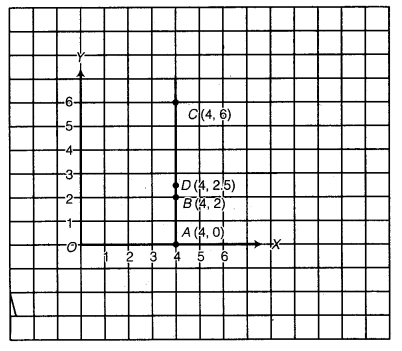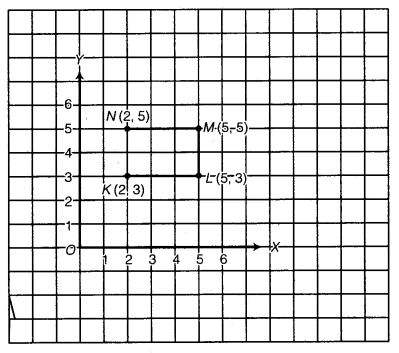# Class 8 Maths NCERT Solutions for Chapter – 15 Introduction to Graphs Ex – 15.2

## Introduction to Graphs

Question 1.
Plot the following points on a graph sheet. Verify if they lie on a line
(a)
A(4, 0), B(4, 2), C(4, 6), D(4, 2.5)
(b) P(1, 1), Q(2, 2), R(3, 3), S(4, 4)
(c) K(2, 3), L(5, 3), M(5, 5), N(2, 5).

Solution:
(a) Draw the x-axis and y-axis. Plot the points A(4, 0), B(4, 2), C(4, 6) and D(4, 2.5) as shown. Clearly, these points lie on a line ABDC.Clearly, these points lie on a line PQRS.
(c) Draw the x-axis and y-axis. Plot the points K(2, 3), L(5, 3), M(5, 5) and N(2, 5) as shown.Clearly, these points do not lie on a line.

Question 2.
Draw the line passing through (2, 3) and (3, 2). Find the coordinates of the points at which this line meets the x-axis and y-axis.

Solution:
Draw the x-axis and y-axis. Plot the points P(2, 3) and Q(3, 2). Join PQ and produce PQ on both sides to meet the x-axis at A and y-axis atB. Clearly, the coordinates of A and B are (5, 0) and (0, 5) respectively.Question 3.
Write the coordinates of the vertices of each of these adjoining figures.

Solution:
Clearly, from the graph the coordinates of points are :
O(0, 0), A(2, 0), B(2, 3) and C(0, 3);
P(4, 3), Q(6, 1), R(6, 5) and S(4, 7);
L(7, 7), M(10, 8) and k(10, 5).

Question 4.
State whether True or False. Correct that are false.
(i)
A point whose x-coordinate is zero and y-coordinate is non-zero will lie on the y-axis.
(ii) A point whose y-coordinate is zero and x-coordinate is 5 will lie on y-axis.
(iii) The coordinates of the origin are (0, 0).

Solution:
(i) True.
(ii) False. Correct statement is : A point whose y-coordinate is zero and x-coordinate is 5 will he on x-axis.
(iii) True.

### Take Your IIT JEE Coaching to Next Level with Examtube

• Mentoring & Teaching by IITians
• Regular Testing & Analysis
• Preparation for Various Engineering Entrance Exams
• Support for School/Board Exams